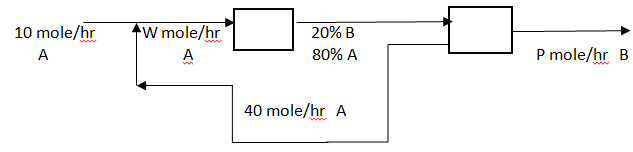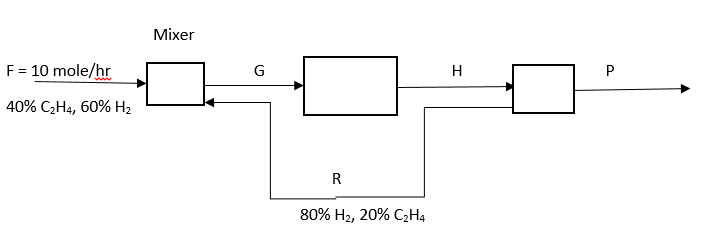# E-PolyLearning

 126. Answer the following question for the given diagram, the reaction is A -> 2B. What is the overall fraction conversion?a. 10% b. 20% c. 80% d. 100%
 127. Answer the following question for the given diagram, the reaction is A -> 2B. What is the single pass fraction conversion?a. 10% b. 20% c. 25% d. 50%
 128. Answer the question for the following diagram. The reaction is C2H4 + H2 -> C2H6, the overall conversion rate of C2H4 is 90%, and the single pass conversion rate is 20%. What is the extent of reaction?a. 3.6 b. 4.8 c. 6.4 d. 7.2
 129. Answer the question for the following diagram. The reaction is C2H4 + H2 -> C2H6, the overall conversion rate of C2H4 is 90%, and the single pass conversion rate is 20%. What are the moles of ethane in products?a. 2.4 b. 3.6 c. 6.4 d. 7.2